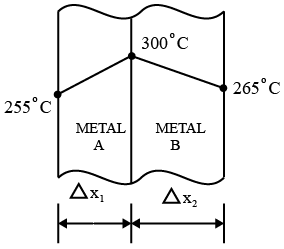# ISRO Scientist or Engineer Mechanical 2011

Instructions

For the following questions answer them individually

Question 11

# A fluid jet is discharging from a 100 mm nozzle and the vena contracta formed has a diameter of 90 mm. If the coefficient of velocity is 0.95, then the coefficient of discharge for the nozzle isQuestion 12

# A fully developed laminar viscous flow through a circular tube has the ratio of maximum velocity to average velocityasQuestion 13

# If the surface tension of water-air interface is 6.073 N/m, the gauge pressure inside a rain drop of 1 mm diameter will beQuestion 14

# A stream function is given by $$(x^2 - y^2)$$. The potential function of the flow will beQuestion 15

# The temperature profile between two metal walls joined together is shown in Fig.From the figure it can be concluded thatQuestion 16

# According to Fourier’s law, amount of heat flow (Q) through the body in unit time is equal toQuestion 17

# Pitch diameter is equal to the product ofQuestion 18

# The tension in the cable supporting a lift moving upwardsis twice the tension when the lift moves downwards. Whatis the acceleration of the lift? |Question 19

# Whirling speed of a shaft coincides with the natural frequency of itsQuestion 20

# Oscillation of a particle is prescribed by the equation $$x = 3 \cos(0.25 \pi t),$$ where t is the time in seconds. The time taken by the particle to move from position of equilibrium to maximum displacement isOR